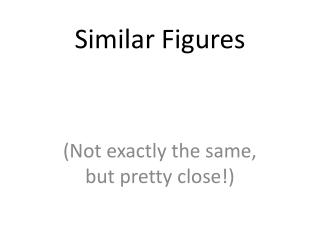# Similar Figures - PowerPoint PPT PresentationDownload PresentationSimilar Figures

Similar FiguresDownload Presentation## Similar Figures

- - - - - - - - - - - - - - - - - - - - - - - - - - - E N D - - - - - - - - - - - - - - - - - - - - - - - - - - -
##### Presentation Transcript

1. Similar Figures (Not exactly the same, but pretty close!)

2. Congruent Figures • In order to be congruent, two figures must be the same size and same shape.

3. Similar Figures • Similar figures must be the same shape, but their sizes may be different.

4. Similar Figures This is the symbol that means “similar.” These figures are the same shape but different sizes.

5. SIZES • Although the size of the two shapes can be different, the sizes of the two shapes must differ by a factor. Factor means multiply, so you must multiply to find scale factor. 4 2 6 6 3 3 2 1

6. SIZES • In this case, the factor is x 2. 4 2 6 6 3 3 2 1

7. SIZES • Or you can think of the factor as 1/2 . 4 2 6 6 3 3 2 1

8. Enlargements • When you have a photograph enlarged, you make a similar photograph. x 3

9. Reductions A photograph can also be shrunk. x 1/4

10. Determine the length of the unknown side. 15 12 ? 4 3 9

11. These triangles differ by a factor of 3, or 1/3. 15 x 1/3 = 5 15 12 ? 4 3 9

12. These dodecagons differ by a factor of 6. ? 2 x 6 = 12 2 24 4

13. Sometimes the factor between 2 figures is not obvious and some calculations are necessary. 15 12 8 10 18 12 12 x ___ = 18 12 18 = 1.5

14. How do you find the scale factor from large to small? To find this missing factor, divide 12 by 18. 15 12 8 10 18 12 18 x __ = 12 2/3

15. When changing the size of a figure, will the angles of the figure also change? ? 40 70 ? ? 70

16. Nope! Remember, the sum of all 3 angles in a triangle MUST add to 180 degrees.If the size of theangles were increased,the sum would exceed180degrees. 40 40 70 70 70 70

17. We can verify this fact by placing the smaller triangle inside the larger triangle. 40 40 70 70 70 70

18. The 40 degree angles are congruent. 40 70 70 70 70

19. The 70 degree angles are congruent. 40 40 70 70 70 70 70

20. The other 70 degree angles are congruent. 4 40 70 70 70 70 70

21. Find the length of the missing side. 50 ? 30 6 8 40

22. This looks messy. Let’s translate the two triangles. 50 ? 30 6 8 40

23. Now “things” are easier to see. 50 30 ? 6 40 8

24. The common factor between these triangles is 5. 50 30 ? 6 40 8

25. So the length of the missing side is…?

26. That’s right! It’s ten! 50 30 10 6 40 8

27. Similarity is used to answer real life questions. • Suppose that you wanted to find the height of this tree.

28. Unfortunately all that you have is a tape measure, and you are too short to reach the top of the tree.

29. Then, measure the length of your shadow. 10 feet 2 feet

30. If you know how tall you are, then you can determine how tall the tree is. 6 ft 10 feet 2 feet

31. The tree must be 30 ft tall. Boy, that’s a tall tree! 6 ft 10 feet 2 feet

32. Similar figures “work” just like equivalent fractions. Equivalent fractions are called a proportion. 30 5 11 66

33. These numerators and denominators differ by a factor of 1/6. 30 x 1/6 5 x 1/6 11 66

34. or 6 30 = 6 x 5 11 66 = 6 x

35. Similar Figures • So, similar figures are two figures that have the same shape, same corresponding angles, and proportionate sides (scale factor).

36. Practice Time!

37. 1) Determine the missing side of the triangle. ? 9 5 3 4 12

38. 1) Determine the missing side of the triangle. 15 9 5 3 4 12

39. 2) Determine the missing side of the triangle. 36 36 6 6 4 ?

40. 2) Determine the missing side of the triangle. 36 36 6 6 4 24

41. 3) Determine the missing sides of the triangle. 39 ? 33 ? 8 24

42. 3) Determine the missing sides of the triangle. 39 13 33 11 8 24

43. 4) Determine the height of the lighthouse. 32 8 2.5 10

44. 5) Determine the height of the car. 7.2 3 5 12

45. THE END!